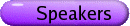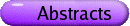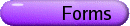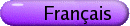Automatic Sequences and Related Topics
Org: Jean-Paul Allouche (Orsay, France) and Jeffrey Shallit (Waterloo)
[PDF]

BORIS ADAMCZEWSKI, CNRS & Institut Camille Jordan (Lyon)
On real numbers generated by finite automata
[PDF]

The b-adic expansion of any rational number is eventually periodic, but how regular or random (depending on the viewpoint) is the b-adic expansion of an irrational algebraic number? It seems that this natural question has been first addressed by Borel in 1950, who made the conjecture that such an expansion should satisfy some of the laws shared by almost all real numbers. Algebraic irrationals are thus expected to be "rather complex" in a probabilistic framework.

In 1965, Hartmanis and Stearns proposed an alternative approach of the notion of complexity of real numbers, by emphasizing the quantitative aspect of the notion of calculability introduced by Turing. According to them, a real number is said to be computable in time T(n) if there exists a multitape Turing machine which gives the first n-th terms of its binary expansion in (at most) T(n) operations. The "simplest" real numbers in that sense, that is, for which one can choose T(n)=O(n), are said to computable in real time. The problem of Hartmanis and Stearns, to which a negative answer is expected, is the following: do there exist irrational algebraic numbers which are computable in real time?

In 1968, Cobham suggested to restrict this problem to a particular class of Turing machines, namely to the case of finite automata. After several attempts, Loxton and van der Poorten finally claimed to have completely solved the restricted problem in 1988. Unfortunately, their proof, which rests on a method introduced by Mahler, contains a rather serious gap, as it has been observed by Becker in 1993.

In this talk, I will report on the recent proof of the Cobham-Loxton-van der Poorten conjecture mentioned above. This result has been obtained in a joint paper with Yann Bugeaud and Florian Luca. A positive answer to a conjecture of Shallit, concerning the Diophantine properties of real numbers generated by finite automata, will also be given. This last result has been obtained in a joint work with Julien Cassaigne.

JEAN-PAUL ALLOUCHE, CNRS, LRI, Bat. 490, F-91405 Orsay Cedex, France
Drawings on the sand in the Vanuatu islands, Indian Kolam, Sierpinski curves and morphisms
[PDF]

People from the Vanuatu islands draw on the sand pictures that have both a ritual and an artistic meaning. These drawings are Eulerian paths on particular graphs (this was explained to us by M. Chemillier: Conférence-Concert at the Musée des Arts d'Afrique et d'Océanie, Paris, Marc Chemillier, Tom Johnson and Daniel Kienzy). The interested reader can read the books of Paulus Gerdes: Une tradition géométrique en Afrique, les dessins sur le sable, Tomes 1, 2 et 3, L'Harmattan.

We show how these drawings are related to Indian pictures called kolam(s), and to a way of representing plane-filling curves (Peano, Hilbert), but also to morphisms of free monoids and finite automata.

We will finally allude to a quickly expanding new field known as ethnomathematics.

JASON BELL, Michigan
Automatic sequences, logarithmic density, and fractals
[PDF]

Let f(n) be an automatic sequence. We consider the logarithmic frequency with which a given letter occurs in this sequence. We completely classify all possible values that can be attained as a logarithmic frequency. Interestingly, the logarithmic frequency is often a ratio of logarithms of natural numbers. Using this motivation, we discuss how to associate a fractal to our automatic sequence whose Hausdorff dimension is equal to the logarithmic frequency with which a given letter occurs.

VALERIE BERTHE, LIRMM, 161 rue Ada, 34392 Montpellier Cedex 5, France
Substitutions, numeration and tilings
[PDF]

The connections between substitutions and numeration systems are numerous and natural: in particular, one can define a numeration system based on finite factors of an infinite word generated by a primitive substitution s, known as the Dumont-Thomas numeration; this numeration system provides generalized radix expansions of real numbers with digits in a finite subset of the number field Q(b), b being the Perron-Frobenius eigenvalue of s. When s is a substitution of constant length l, one recovers the classic l-adic numeration. A characteristic example is given by the Fibonacci substitution 1® 12, 2® 1 and by the Fibonacci numeration (where non-negative integers are represented thanks to the usual Fibonacci recurrence with digits in {0,1} and no two one's in a row allowed).

It is possible, generalizing Rauzy's and Thurston's constructions, to associate in a natural way either with a Pisot number b (of degree d) or with a Pisot substitution s (on d letters) some compact basic tiles that are the closure of their interior, that have non-zero measure and a fractal boundary. We know that some translates of these prototiles under a Delone set G (provided by b or s) cover Rd-1; it is conjectured that this multiple tiling is indeed a tiling (which might be either periodic or self-replicating according to the translation set G). This conjecture is known as the Pisot conjecture.

The aim of this lecture is to state for Pisot substitutions a finiteness property in terms of the Dumont-Thomas numeration which is a sufficient condition to get a tiling.

This is joint work with A. Siegel.

JAMES CURRIE, University of Winnipeg, 515 Portage Ave., Winnipeg, MB R3B 2E9
On k-avoidability of abelian powers and patterns
[PDF]

We give results and interconnections concerning

(1) avoiding abelian r-powers (r integer or fractional);
(2) avoiding long abelian squares and cubes;
(3) abelian k-avoidability of binary patterns.

ANNA FRID, Sobolev Institute of Mathematics SB RAS
On complexity of infinite permutations
[PDF]

Let us say that two sequences of pairwise distinct reals ...,a1,a2,... and ...,b1,b2,... defined on the same set S (which can be finite, or equal to N or Z) are equivalent if for all i,j Î S we have ai < aj if and only if bi < bj. An equivalence class of sequences on S will be called an (S-)permutation. An S-permutation can be also interpreted as a linear ordering of S. A permutation [`(a)] having a representative a = ... a1,a2,... is called t-periodic if for all i,j such that i,j,i+t,j+t Î S we have ai < aj if and only if ai+t < aj+t. An N-permutation is called ultimately t-periodic if the periodicity property holds for all i,j ³ n0 for some n0.

Surprisingly, for all t ³ 2 there exist infinitely many t-periodic Z-permutations. We characterize them and give a way to code each of them.

Then we define complexity f[`(a)](n) of a permutation [`(a)] as the number of permutations (i.e., equivalence classes) [`(ak,ak+1,...,ak+n-1)]. Analogously to the subword complexity of words, this function is non-decreasing, and we have:

Theorem 1 Let [`(a)] be a Z (N-)permutation; then f[`(a)](n) £ C if and only if [`(a)] is periodic (ultimately periodic).

However, other properties of subword complexity cannot be directly extended to complexity of permutations: in particular, one-sided and two-sided infinite permutations have different minimal complexity.

Theorem 2 For each unbounded growing function g(n) there exists a not ultimately periodic N-permutation [`(a)] with f[`(a)](n) £ g(n) for all n ³ n0. On the other hand, for each non-periodic Z-permutation [`(a)] we have f[`(a)](n) ³ n-C for some constant C which can be arbitrarily large.

This is a joint work with D. G. Fon-Der-Flaass.

KIRAN KEDLAYA, MIT, Dept. of Mathematics, Room 2-165, 77 Mass. Ave., Cambridge, MA 02139, USA
Finite automata and algebraic extensions of function fields
[PDF]

A classic theorem of Christol characterizes those power series over a finite field which are algebraic over the rational function field, as those whose coefficients can be generated by a finite automaton. However, not all elements in the algebraic closure of the function field can be represented by ordinary power series; those lying in wildly ramified extensions can only be represented using "generalized power series" in the sense of Hahn-Malcev-Neumann. We describe a natural extension of Christol's theorem, characterizing the generalized power series which are algebraic over the rational function field essentially as those whose coefficients are generated by some finite automaton.

DALIA KRIEGER, Waterloo

XAVIER LE BRETON, LRI, Bâtiment 490, Université Paris-Sud, F-91405 Orsay Cedex, France
Tyszka-characterisation property on automatic sequences
[PDF]

Our work is based on Tyszka's article: A discrete form of the theorem stating the non-existence of non-identical field endomorphisms of R. Tyszka studies a characterisation property on a field and proves that this property is equivalent to algebricity on R and Qp. Amazingly, the same property is equivalent to rationality on C.

We introduce a similar characterisation property which is equivalent to algebricity on normed and complete fields of characteristic 0. Our main interest is to study this new property on normed and complete integral domains of strictly positive characteristic and particularly on Fp [[X]].

We will underline the essential part played by Cartier's operators in these structures. By adding a hypothesis (related to Cartier's operators) on these integral domains, we will be able to prove an equivalency theorem for algebricity.

BRENDAN LUCIER, University of Waterloo, 200 University Ave. W., Waterloo, Ontario N2L 3G1
Proximity Inversion Functions: A Numeration Approach
[PDF]

We consider functions mapping non-negative integers to non-negative real numbers such that a and a+n are mapped to values at least [ 1/(n)] apart for all a and n. We use a novel method to construct such a function using a numeration system and combinatorics on words. We conjecture that the supremum of the generated function is optimal and pose some unsolved problems.

MORTEZ MOHAMMAD-NOORI, Universite Paris XI; Bat. 490, LRI, Universite Paris XI, 91405 Orsay Cedex, France
Dejean's conjecture and Sturmian words
[PDF]

Dejean conjectured that the repetition threshold of a k-letter alphabet is k/(k-1), k ¹ 3,4. The history goes back to Thue's famous papers of 1906 and 1912. Values of the repetition threshold for k < 5 were found by Thue, Dejean and Pansiot. Moulin-Ollagnier attacked Dejean's conjecture for 5 £ k £ 11. Building on the work of Moulin-Ollagnier, here we propose a method to decide whether a given Sturmian word with quadratic slope validates the conjecture for a given k. We develop this method into a search algorithm for verifying the conjecture for a given k. An implementation of our algorithm gives suitable Sturmian words for 7 £ k £ 14. Moreover, we prove that for k=5, no suitable Sturmian word exists.

Binary words, avoidable powers, and the constant 7/3
[PDF]

In recent years, the fraction 7/3 has appeared several times as a threshold for various properties of binary words. Shur showed that the bi-infinite overlap-free words are exactly the bi-infinite 7/3-power-free words. Karhumäki and Shallit showed that Restivo and Salemi's factorization theorem for overlap-free binary words holds for 7/3-power-free binary words as well. They also showed that the threshold between polynomial growth and exponential growth for k-power-free binary words is k = 7/3. Kolpakov, Kucherov, and Tarannikov showed that k = 7/3 is also a threshold for the minimal letter density in k-power-free binary words. We present here a generalization of a result by Séébold by showing that the only infinite 7/3-power-free binary words that can be obtained by iterating a morphism are the Thue-Morse word and its complement. Further, the constant 7/3 is best possible.

MICHEL RIGO, University of Liege, Department of Mathematics, Grande Trave 12 (B37), B-4000 Liege, Belgium
Abstract numeration systems, additive functions and automatic sequences
[PDF]

Abstract numeration systems are a natural generalization of positional numeration systems whose set of representations of all the integers is a regular language. They were introduced five years ago by P. Lecomte and myself  and are defined in the following way. Consider any infinite regular language L over a totally ordered alphabet (A, < ). An abstract numeration system is thus simply given by the triple S = (L,A, < ). The words of L can be enumerated with respect to the genealogical ordering induced by the total ordering of A. We therefore have a one-to-one correspondence between the set of nonnegative integers and the language L. We say that the (n+1)-st word of L is the S-representation of the integer n.

In this general setting, various notions arising in the study of "classical" numeration systems (p-ary system or systems built over a strictly increasing sequence of integers like the Fibonacci system) can be generalized and may give rise to new phenomena. In particular, if we consider a finite automaton with output fed with the S-representations of the successive integers, we obtain a notion of "generalized" automatic sequence or S-automatic sequence , .

In this talk, I will mainly focus on a problem related to completely additive functions defined over the alphabet A and taking real values, i.e., f(a1¼ak) = f(a1)+¼+f(ak) for any finite word a1¼ak over A. With P. Grabner, we have studied, in the framework of abstract numeration systems, as a first step the asymptotic behaviour of the summatory function of additive functions  and as a second step the distribution of such functions . The obtained results can naturally be applied to study the frequency of a symbol appearing in a generalized automatic sequence (assuming that the frequency exists) .

## References


P. Grabner, M. Rigo, Additive functions with respect to numeration systems on regular languages. Monatsh. Math. 139(2003), 205-219.


, Distribution of additive functions with respect to numeration systems on regular languages. Submitted.


P. B. A. Lecomte and M. Rigo, Numerations systems on a regular language. Theory of Comput. Syst. 34(2001), 27-44.


S. Nicolay and M. Rigo, About the density of generalized automatic sequences. Submitted.


M. Rigo, Generalization of automatic sequences for numeration systems on a regular language. Theoret. Comput. Sci. 244(2000), 271-281.


M. Rigo and A. Maes, More on generalized automatic sequences. J. Autom. Lang. Comb. 7(2002), 351-376.

Various preprints are available on my homepage http://www.discmath.ulg.ac.be.

KALLE SAARI, University of Turku, University of Waterloo
On the frequency of letters in morphic sequences
[PDF]

In papers that appeared in 1975 and 1976, Michel studied the existence of the asymptotic frequency of letters in a morphic sequence. He proved that if a sequence is primitive, then the letter frequency exists. This is not, however, optimal; there are nonprimitive morphic sequences for which the letter frequency exists. In this talk, we present some new results concerning the existence of the frequency of letters in morphic sequences. In particular, we outline a proof of a result that the frequency of letters exists in any pure binary morphic sequence.